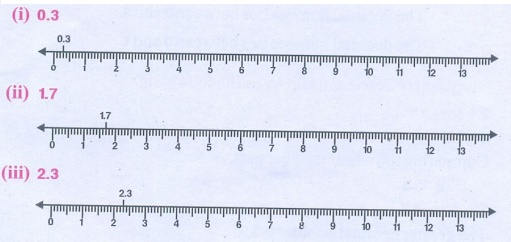Home | | Maths 7th Std | Representing Decimal Numbers on the Number Line

# Representing Decimal Numbers on the Number Line

We have already learnt to represent fractions on a number line. Now let us see the representation of decimal numbers on a number line.

Representing Decimal Numbers on the Number Line

We have already learnt to represent fractions on a number line. Now let us see the representation of decimal numbers on a number line. Consider the decimal number 0.8.

That is there are 8 tenths (8/10). We know that 0.8 is more than 0 and less than 1. Let us divide the unit length into ten equal parts and take 8 parts as shown below.

Draw a number line and divide the intervals into ten equal parts. Now 0.8 lies between 0 and 1.Can we represent 1.4 on a number line? Let us see now. 1.4 has one and four tenths in it. So it lies between 1 and 2. It is marked on the number line as shown below.Try these

1. Mark the following decimal numbers on the number line.

(i) 0.3

(ii) 1.7

(iii) 2.32. Identify any two decimal numbers between 2 and 3.

The decimal numbers between 2 and 3 are 2.4, 2.7.

3. Write any decimal number which is greater than 1 and less than 2.

The decimal number which is greater than 1 and less than 2 is 1.5.

Tags : Number System | Term 2 Chapter 1 | 7th Maths , 7th Maths : Term 2 Unit 1 : Number System
Study Material, Lecturing Notes, Assignment, Reference, Wiki description explanation, brief detail
7th Maths : Term 2 Unit 1 : Number System : Representing Decimal Numbers on the Number Line | Number System | Term 2 Chapter 1 | 7th Maths